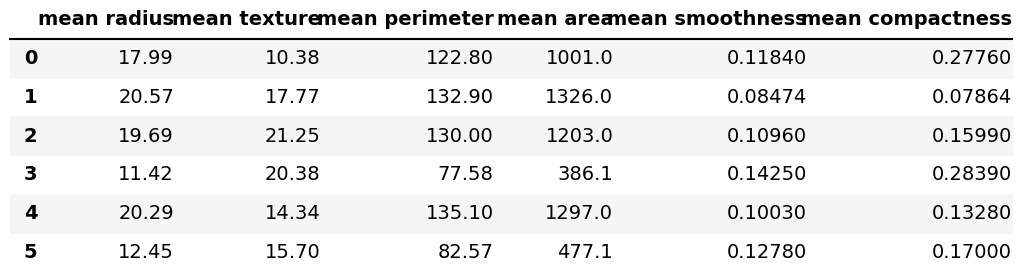# Scatterplot of PCA in Python (2 Examples)

In this tutorial, we will show how to visualize the results of a Principal Component Analysis (PCA) via scatterplot in Python.

The table of content is as follows:

This page was created in collaboration with Paula Villasante Soriano and Cansu Kebabci. Please have a look at Paula’s and Cansu’s author pages to get further information about their academic backgrounds and the other articles they have written for Statistics Globe.

Let’s move on to defining example data and importing relevant libraries!

## Sample Data & Add-On Libraries

The first step in this tutorial is importing the libraries to be used in the analysis. You can do this by running the lines of code below:

```import numpy as np
import pandas as pd
import matplotlib.pyplot as plt
import seaborn as sns
from sklearn.preprocessing import StandardScaler
from sklearn.decomposition import PCA```

Now it’s time to load our data. For the demonstration, we will use the breast cancer data set, from the scikit-learn library. This data is composed by a data matrix, which has 569 rows and 30 columns, representing 569 samples and 30 features, and a classification target, which contains the information of the type of tumor for each sample: malignant or benign.

The load() function will help us to load the data set, and the DataFrame() function of pandas will help to convert our data into a pandas DataFrame.

```b_cancer = load_breast_cancer()

df = pd.DataFrame(data=b_cancer.data,
columns=b_cancer.feature_names)```

We can have a quick view of the dataset using the `.iloc[]` method and the head() function as follows:

`df.iloc[:, 0:6].head(6)`Now, let’s go straight to the PCA.

## Data Standardization

To perform the PCA, we need to standardize the data first. If you wonder why to do so, visit our tutorial PCA Using Correlation vs Covariance Matrix. To achieve this, we will use the StandardScaler() class to transform our data, as shown below.

```scaler = StandardScaler()

scaler.fit(df)

Bcancer_scaled = scaler.transform(df)```

## Principal Component Analysis

Now, we can compute the PCA and transform our data into its new dimensions formed by the principal components. In this example, will choose 2 components for illustrative purposes. If you want to learn more about choosing the optimal number of components, please check our tutorial: How to Choose the Optimal Number of Components.

```pca = PCA(n_components=2)

pca.fit(Bcancer_scaled)

pca_bcancer = pca.transform(Bcancer_scaled)```

In order to visualize the results of the PCA on a scatterplot, we will extract the first two components to be shown:

```PC1 = pca_bcancer[:,0]
PC2 = pca_bcancer[:,1]```

As early said, the data has a classification target for the breast cancer type. Therefore, coloring the data by its target might be interesting, which is presented as 0 for malignant and 1 for benign. In order to do this, we will create a DataFrame that contains the two principal components, the classification target, and the target labels.

We will use a for loop to create a list named `labels[]` which will contain the labels for each type of breast cancer. Then we will use the zip() and list() functions to create the data for our DataFrame:

```labels=[]

for points in b_cancer.target:
labels.append(b_cancer.target_names[points])
zipped = list(zip(PC1,
PC2,
b_cancer.target,
labels))

pc_df = pd.DataFrame(zipped,
columns=['PC1',
'PC2',
'Target',
'Label'])## Example 1: Scatterplot of PCA Using Matplotlib

To create our scatterplot by Matplotlib, we will split our data into 4 data series based on the combinations of the two principal components and the classification targets.

```PC1_m = pc_df.loc[pc_df["Target"] == 0,
"PC1"]
PC2_m = pc_df.loc[pc_df["Target"] == 0,
"PC2"]

PC1_b = pc_df.loc[pc_df["Target"] == 1,
"PC1"]
PC2_b = pc_df.loc[pc_df["Target"] == 1,
"PC2"]```

Then we will use the scatter() function to create our scatterplot using the inputs defined above and the arguments `c="blue"` and `label="Malignant"` for the malignant type of tumor, and `c="orange"` and `label="Benign"` for the benign type.

```fig = plt.figure(figsize=(12,7))

ax.scatter(PC1_m,
PC2_m,
c="blue",
label="Malignant")

ax.scatter(PC1_b,
PC2_b,
c="orange",
label="Benign")

ax.legend(title="Label")

plt.title("Figure 1",
fontsize=16)
plt.xlabel('First Principal Component',
fontsize=16)
plt.ylabel('Second Principal Component',
fontsize=16)```Figure 1 shows a scatterplot colored by the type of breast cancer using the Matplotlib package.

## Example 2: Scatterplot of PCA Using Seaborn

We can also use the seaborn package to create our scatterplot. In order to do that, we can simply use the scatterplot() function by plugging the defined principal components: PC1 and PC2, and add the target label using the `hue="label"` argument, which helps with plotting the points in orange or blue depending on the type of cancer.

```plt.figure(figsize=(12,7))

sns.scatterplot(data=pc_df,
x="PC1",
y="PC2",
hue="Label")

plt.title("Figure 2",
fontsize=16)
plt.xlabel('First Principal Component',
fontsize=16)
plt.ylabel('Second Principal Component',
fontsize=16)```Figure 2 shows a scatterplot colored by the type of breast cancer using the seaborn package.

As you can see, we can obtain the same output using either Matplotlib or the seaborn package. If you are also interested in visualizing the PCA results in 3D, see our tutorial: 3D Plot of PCA in Python.

## Video, Further Resources & Summary

Do you need more explanations on the steps and application of a Principal Component Analysis in Python? Then you should have a look at the following YouTube video of the Statistics Globe YouTube channel.

Check some other tutorials on Statistics Globe:

This post has shown how to draw a scatterplot based on a PCA in Python. In case you have further questions, you may leave a comment below.

Subscribe to the Statistics Globe Newsletter